# Kramers-Kronig and Ellipsometry Techniques

## Introduction

The are two main schemes for optically investigating a material's complex dielectric properties and index of refraction. The first technique is a Kramers-Kronig analysis of the material's reflectivity or absorption over the full optical spectrum. The second technique is ellipsometry which involves measuring slight changes to the polarization vector of light reflected from a sample. Here we will introduce both techniques.

## Kramers-Kronig Optical Reflectivity Analysis

The Kramers-Kronig relations were discovered independently by Hendrik Anthony Kramers in 1927  and Ralph de Laer Kronig in 1926 . Broadly speaking, these relations describe a fundamental mathematical connection between the real and imaginary parts of certain analytic functions. In practice, these relations are used to investigate the in-phase and out-of-phase responses of linear systems due to various excitations. Perhaps the most common application of the Kramers-Kronig relations is found in optical spectroscopy. (They are also heavily used in high energy particle physics, acoustics and statistical mechanics.) The K-K relations connect the real and imaginary parts of a material's index of refraction. This connection allows one to use full-spectrum transmission or reflection data to calculate the real and imaginary parts of a material's refraction index, which is the complex square root of the dielectric constant.

## Reflectivity and the Dielectric Constant

The need for Kramers-Kronig analysis arises from the fact that optical reflectivity is a consequence of both the real and imaginary parts of a material's index of refraction and dielectric constant. This is evident in the normal incidence reflectivity (from air) equation seen below.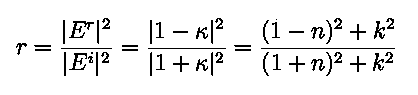Here 'r' is reflectivity at normal incidence, 'Er' is the reflected electric field, 'Ei' is the incident electric field, &kappa is index of refraction, and 'n' and 'k' are the real and imaginary parts of the index of refraction as see below. The dielectric constant '&epsilon' is the square of the index of refraction.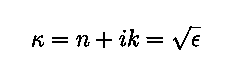At first look, there seems to be no way to use 'r' to calculate the index of refraction components 'n' and 'k'. The Kramers-Kronig relations give a solution to this inverse problem. They connect the real and imaginary parts of the index of refraction, eliminating the free variable. The numerics, however, can be rather tricky, and most research groups seem to have slightly different implementations of the procedure.

## The Kramers-Kronig Relations

The Kramers-Kronig relations for Optical Spectroscopy are given below.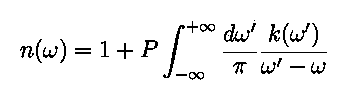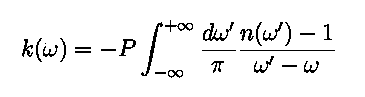We have adopted the same notation used in the popular Solid State Physics textbook by Ashcroft and Mermin . 'P' denotes the Cauchy Principal Value. The first of these equations is even in &omega while the second is odd, allowing us to recast the integrals in the following form to avoid unwieldy negative frequencies .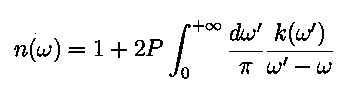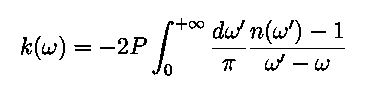A full Kramers-Kronig analysis requires a complete optical spectrum of either the reflection or absorption coefficients, as the integrals suggest. The absorption method is more limited because the thickness of the sample must be known very accurately, and it must be less than the wavelength of light used. With either scheme, an accurate interpolation or extrapolation scheme is often needed to fill in unmeasured parts of the spectrum. Also, the samples should be prepared very carefully so as to avoid surface roughness effects which may create anamolous data.

For information on the actual implementation and numeric techniques used in Kramers-Kronig analysis, see the recent text by by Lucarini et al. "Kramers-Kronig Relations in Optical Materials Research" . They have comprehensively assembled various different basic and modified Kramers-Kronig schemes and present actual optical reflectivity data, resulting calculations of the dielectric constants, and the computer codes used. Also, it should be noted that the derivation of the Kramers-Kronig relations, though not presented here, is rich in complex mathematics and principles of causality. Lucarini's text also does a masterful job of presenting these concepts.

## Ellipsometry

Ellipsometry is an alternate technique for optically determining a material's index of refraction. Ellipsometry is more commonly used for thin films, and it involves measuring the ellipticity induced in a polarized beam of light reflected off a sample surface. The complex refraction index of the material can be indirectly deduced from this information. As a sanity check, the real and imaginary parts of this index should satisfy the Kramers-Kronig relations.

## Experimental Setup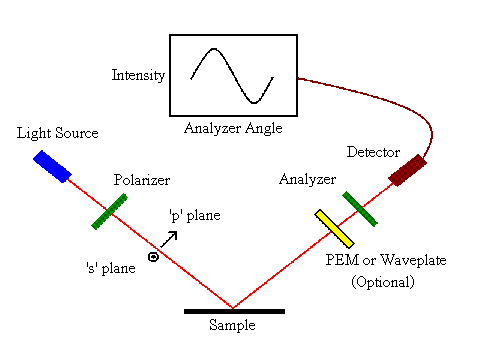Fig. 1: A typical ellipsometry experimental setup.

A typical ellipsometry setup is pictured in figure 1. A high grade polarizer filters a beam from a light source into the 's' or 'p' plane relative to the sample. The polarized light then hits a sample, inducing ellipticity based on the dielectric properties of the material. The resulting light is then filtered through another polarizer known as the analyzer, and then an intensity measured in a photodetector. Rotating the analyzer produces a sinusoidal change in intensity as seen in figure 1. Other experiment configurations may include a quarter waveplate or a combination of a lock-in amplifier and photoelastic modulator.

## Measurement and Analysis

The actual quantity measured an ellipsometry experiment is the intensity of light at the detector as a function of the analyzer angle. This can be used to determine rp and rs, the components of the polarization in the 'p' and 's' planes. The ratio rp/rs and the phase difference between rp and rs determine the polarization ellipticity of the beam. These two quantities can then be matched to numeric predictions given by the Fresnel Equations to indirectly deduce the real and imaginary parts of the index of refraction. Again, these results (if obtained over the full spectrum) should satisfy the Kramers-Kronig relations.

© 2007 J. S. Brockman. The author grants permission to copy, distribute and display this work in unaltered form, with attribution to the author, for noncommercial purposes only. All other rights, including commercial rights, are reserved to the author.

## References

 H. A. Kramers, "La diffusion de la Lumiere par les Atomes," Atti del Congresso Internazionale dei Fisici 2, 545 (1927).

 R. de L. Kronig, "On the theory of Dispersion of X-Rays," J. Opt. Soc. Am. 12, 547 (1926).

 N. W. Ashcroft and N.D. Mermin, Solid State Physics, Appendix K (Holt, Rinehart and Winston, 1976).

 J. D. Jackson, Classical Electrodynamics, 2nd edition, Sec. 7.10 (Wiley, 1975).

 V. Lucarini, J. J. Saarinen, K.-E. Peiponen, and E. M. Vartiainen, Kramers-Kronig Relations in Optical Materials Research, (Springer, 2005).